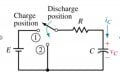# RC circuit/Laplace transform/homework problem

#### Saitron

Joined Mar 20, 2020
13
Hello i have a question about a RC circuit. Can i represent with Laplace Transform the discharging of RC curcuit and how i get the transfer funtion to represent the free responce of the system

#### MrAl

Joined Jun 17, 2014
10,871
Are you talking about an RC low pass filter?
The simplest way is to replace the capacitor with its impedance and do the same as you would as with a resistive voltage divider. It takes about 1 minute to do this way.

#### Saitron

Joined Mar 20, 2020
13
Yes it is very efective to find the transfer function with the impedance. My problem is that when i take the the diagram of the discharging equation(differential equation) i get exp(-x/RC) graph everything is ok here, but when i try to get the free response of this system with the transfer function i get the opposite of this function exp(x). Is this right?

#### MrAl

Joined Jun 17, 2014
10,871
Yes it is very efective to find the transfer function with the impedance. My problem is that when i take the the diagram of the discharging equation(differential equation) i get exp(-x/RC) graph everything is ok here, but when i try to get the free response of this system with the transfer function i get the opposite of this function exp(x). Is this right?
Can you show the circuit you want to analyze? It is best to see the circuit when talking about these details.

#### WBahn

Joined Mar 31, 2012
29,469
Yes it is very efective to find the transfer function with the impedance. My problem is that when i take the the diagram of the discharging equation(differential equation) i get exp(-x/RC) graph everything is ok here, but when i try to get the free response of this system with the transfer function i get the opposite of this function exp(x). Is this right?
You need to show the problem you are trying to solve and your best attempt to work it. Otherwise we have very little to go on and are highly likely to end up talking at cross purposes because our crystal balls didn't show us your circuit as we were guessing.

#### MrAl

Joined Jun 17, 2014
10,871
Yeah mine broke about a month ago and i have been meaning to get it fixed but the shop down the street told me the wizard that usually does that stuff is out of town.However, i think i have an idea what he might have been talking about...

Yes it is very efective to find the transfer function with the impedance. My problem is that when i take the the diagram of the discharging equation(differential equation) i get exp(-x/RC) graph everything is ok here, but when i try to get the free response of this system with the transfer function i get the opposite of this function exp(x). Is this right?
@Saitron, yes that is what you get e^-at and then you have to solve for the constant using the initial conditions. You then get the full result you are probably expecting. So there are really two steps involved. One to solve the DE as you have done and then another to apply the initial conditions.
Do you know how to do this?

#### Saitron

Joined Mar 20, 2020
13
Hahaha don't troll me dudes.. i guess i would be more helpful if i show you the circuit. Ok let's go to the topic. I have this circuit and i charge it with 100V, R=1, C=3F, then i disconect the source and let the capacitor discarge. I know that the function on the capacitor is V=Voe^(-t/RC) and i can get the graph. My problem is how to get the transfer function of this because the graph of the differential funtion is kind of exp(-x) and the graph of the transfer function is exp(x). Is it this right#### MrAl

Joined Jun 17, 2014
10,871
Hahaha don't troll me dudes.. i guess i would be more helpful if i show you the circuit. Ok let's go to the topic. I have this circuit and i charge it with 100V, R=1, C=3F, then i disconect the source and let the capacitor discarge. I know that the function on the capacitor is V=Voe^(-t/RC) and i can get the graph. My problem is how to get the transfer function of this because the graph of the differential funtion is kind of exp(-x) and the graph of the transfer function is exp(x). Is it this rightView attachment 203128
Well the exponent is always negative for this.
Vc(t)=Vc0*e^(-t/T)
where T is the time constant and Vc0 is the initial voltage of the capacitor.
I dont know how you get e^x but that's not right so show how you arrived at that solution.